# How to use the FV formula in Google SheetsWritten by
Holly MeikleKeeping finances in order is a lot easier when you use spreadsheets. Google Sheets offers a variety of intelligent functions that can be used to help calculate useful finance-related values quickly and easily. If you’re looking to calculate the value of your investments in the future, then the FV formula in Google Sheets is the function you need.

The FV formula can calculate the future value of your investments for periodic payments with fixed interest rates. For example, if you add a monthly figure to your business savings account, you can forecast your total value at a later date by using the FV formula, which takes into account the compound interest. Not only does the FV formula streamline the entire calculation progress, but it also guarantees accurate results.

Let’s explore in more detail what the FV formula is and how you can use it effectively in Google Sheets.

## What is the FV formula in Google Sheets?

The FV formula stands for ‘Future Value’. It’s usually used to calculate the future value of any investments with compound interest. In order to use the FV formula, you need to have periodic payments (these can be annual, quarterly, weekly, etc.), and a fixed interest rate. You can also use the FV formula to calculate lump sums with compound interest too.

Let’s take a look at the syntax of the FV formula and its parameters.

### Syntax

The FV formula uses the following syntax to calculate your future value:

=FV(rate, number_of_periods, payment_amount, [present_value], [end_or_beginning])
• rate: the fixed amount of interest over time.
Interest rates are always annual. If you want to calculate the interest rate for more frequent payments (quarterly or monthly), make sure to divide the rate accordingly, i.e. for a monthly interest rate, divide by 12.
• number_of_periods: the number of periodic payments that you are going to make
• payment_amount: the constant amount of money that you pay for each period.
• This will be the type of payment (weekly (52), monthly (12), quarterly (4) multiplied by the length of time, i.e. monthly payment over 3 years: 12*3 = 36 periods.

• [present_value]: [OPTIONAL – 0 by default] the current value of the investment.
• [end_or_beginning]: [OPTIONAL – 0 by default] a value of 0 indicates that you are making the payments at the end of each period. A value of 1 specifies that we are making payments at the beginning of each payment period.

### How to use the FV formula in Google Sheets

Now that you understand what the FV formula is and how it works, let’s go ahead and apply it to some real-life examples.

#### 1. Use the FV formula to calculate the future value of an investment

Let’s say I want to add \$5,000 at the beginning of every month into my business savings account over the course of a year. The annual interest rate of my account is 3%.

The screenshot below shows the values of each aspect that we need to substitute into the FV formula.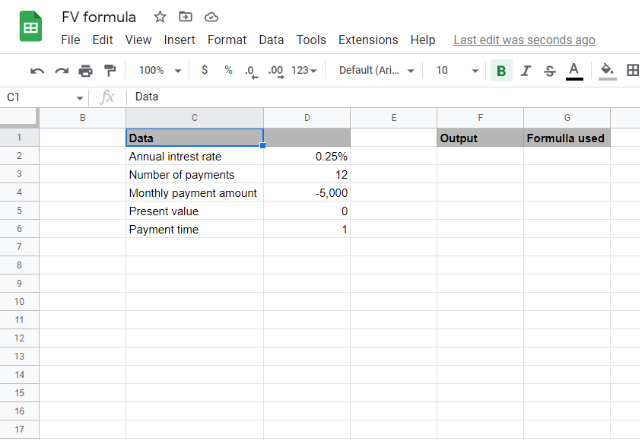Please note: As I am paying monthly, the annual interest rate needs to be divided by 12. As I am paying monthly over one year, the number of payments is 12.

To calculate the future value of my total investment after a year, I’d input the following formula:

=FV(0.25%,12,-5000,0,0)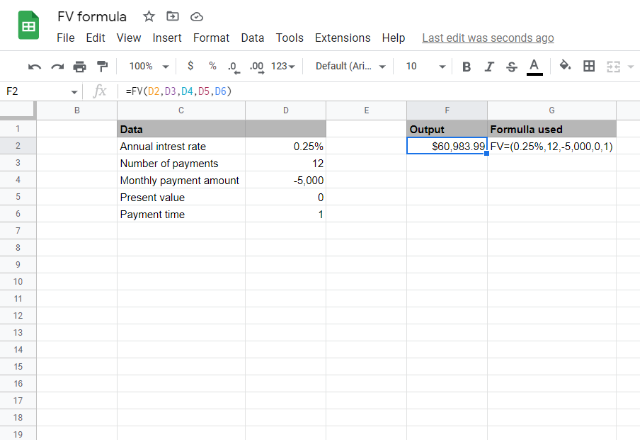As you can see, my total investment over 12 months would be a total of \$60,983.99. You can either input the numbers directly into the cell, or you can reference the cells containing each value within the formula.

#### 2. Use the FV formula to calculate the future value of a lump-sum payment

You can also calculate the future value of a one-time investment payment over a period of time.

Let’s say I have inherited \$120,000 that I want to keep in a savings account over the next 10 years. The annual interest rate is 1%.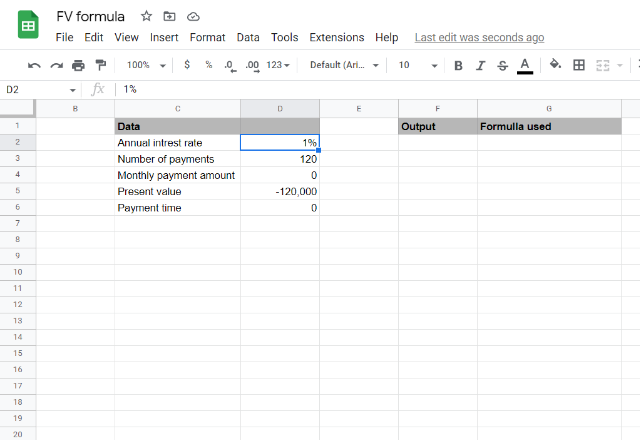Please note: As this is a lump sum going into the savings account, it’s important to remember that the [present_value] is negative.

To calculate the future value of this lump-sum, I’d input the following formula:

=FV(1%,120,0,-120000,0)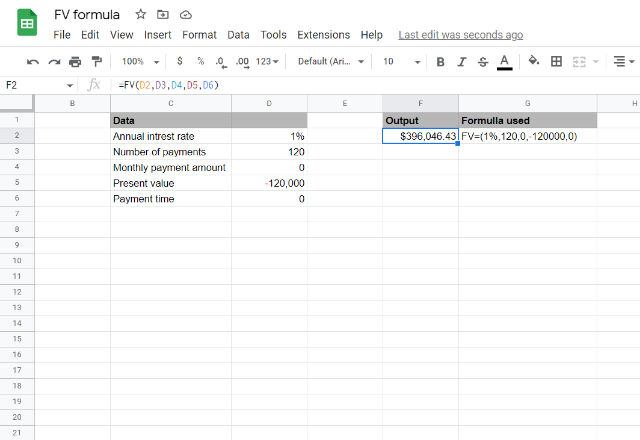As you can see, I will end up with \$396,046.43 at the end of 10 years!

#### 3. Use the FV formula to calculate loan payback

You can also use the FV formula to calculate whether you’ll be able to pay off a loan in a certain amount of time. You can do this by calculating whether an investment of a certain amount over a certain period of time would leave you with a positive balance. If the value is positive, you will successfully pay back the loan in time. If the final value is negative, you will need to increase the value of your payments or the number of payment periods.

Let’s say I need to pay back a business loan of \$100,000. I want to pay it back over 3 years, paying a fee of \$12,000 at the end of each quarter. The annual interest rate of the loan is 5%.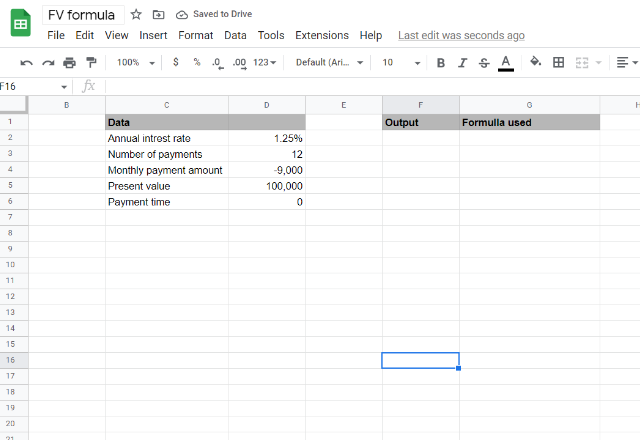Please note: As I am paying back at the end of each quarter, I need to divide the annual interest rate by 4, which gives me 1.25%. As I’ll be paying back the loan over 3 years, the payment period is 12 (4 quarters multiplied by 3 years).

To calculate whether I can pay of the loan in 3 years, I’d input the following formula:

=FV(1.25%,12,-9000,100,000,0)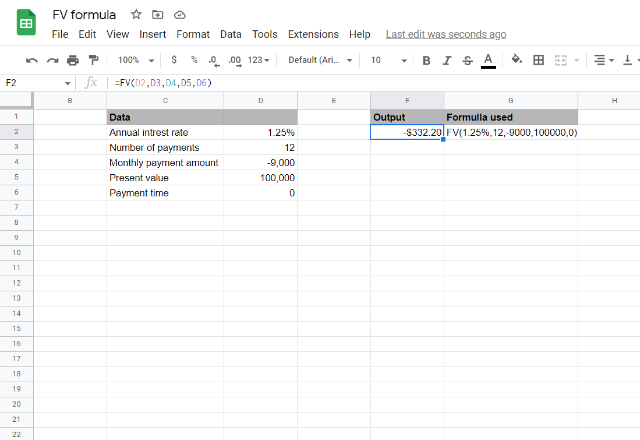As you can see, the value returned is negative, meaning that I won’t be able to pay back the loan over the course of 3 years. However, if I swap my payments to the beginning of the month, the FV formula shows that I can pay off the loan over 3 years – see below.### FV function in Google Sheets

And there you have it! The FV formula is the most accurate and efficient function to use when calculating the future value of any investment or to help calculate whether you can pay back a loan. It’s important to remember that, in order for the FV formula to work, you must have payments that are at even intervals. Furthermore, you must also adapt the interest rate to the payment frequency.

If you’re interested in using more finance-related formulas in Google Sheets, why not take a look at How to use the IRR formula in Google Sheets? Alternatively, check out some related blog posts below!

Editor’s note: This is a revised version of a previous post that has been updated for accuracy and comprehensiveness.